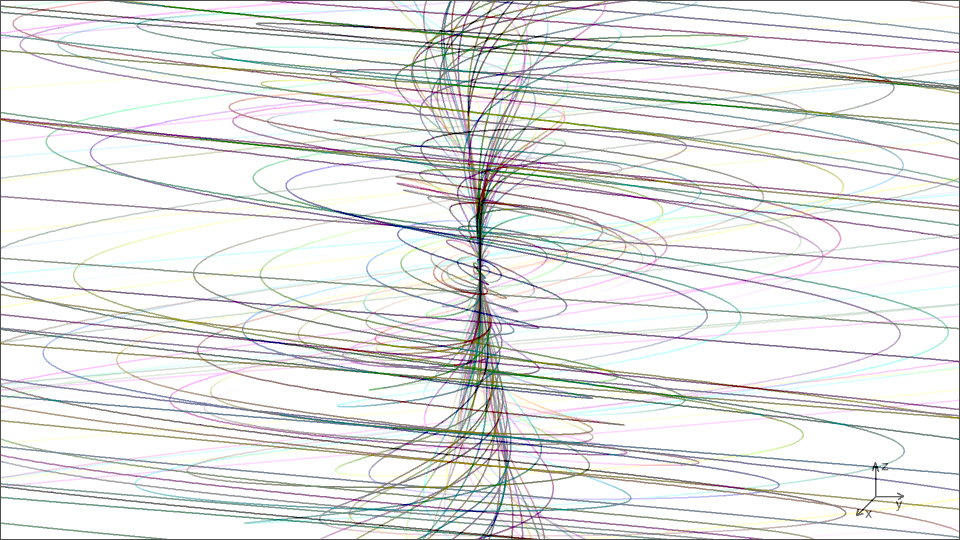horn torus entityx = r·(1 − cosφ)·cos(φ2/2π)
y = r·(1 − cosφ) · sin(φ2/2π)
z = r · sinφr = r1·φ / 2π for r > r1
φ = 2π when r = r1 *)
φ < 2π doesn't occur !

φ is the poloidal horn torus angle, r in the graphic above is quite to scale
(increase is faster than in the 2nd graphic, r < r1), depicted is the section
between 10 revolutions per 1 rotation  and ∼7 rotations per 1 revolution
(for explanation click image)

*) r1 signifies the standard dynamic horn torus, the 'μ-sterious' mirror between 'infinite outer world', consisting of electrons and photons, and the 'small inner world' of hadrons and infinitesimal infinity.

Important note 1 in this context: the widely unknown infinitesimal infinity has the same cardinality as our known large universe (i.e. nuclei comprise whole universes). It's worth to think about that!

Note 2, equally imperative: horn torus depictions are allegoric only, their purpose is to symbolise mathematical particle properties - they don't occur as objects in our familiar 3-dimensional space.

Dynamic variation of poloidal revolution and toroidal rotation, combined with alteration of size, can be expressed smartly by complex and hypercomplex numbers (quaternions, octonions, ...) - but

to describe the whole dynamics as abstract physical processes, including the interaction of intertwined nested horn tori, seems to be a task, which is not manageable by conventional mathematics,

so, in this regard, the author is not ready yet :-(